# Barendregt's Lambda Cube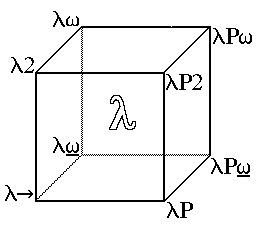The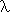cube is a way of presenting the fine structure of Coquand's Calculus of Constructions relating that system to a variety of other typed lambda calculi. This idea is due to Henk Barendregt, and is presented in his Introduction to Generalised Type Systems which is my principle source in preparing this material.

The eight systems at the vertices of the cube are obtained by extending the simply typed lambda calculus (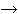) at the origin of the cube along one or more of the axes of the cube. The cube has six faces.

To understand the following presentation of the lambda cube you must first refer to the page on Pure (or generalised) Type Systems. The deductive system given there is parameterised by a triple (S,A,R), in which S is a set of sorts, A is a set of axioms and R is a set of triples of sorts. The relation R determines which products are available in the system.

For the systems on the lambda cube the sorts are always {*,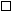} (it may be helpful to think of these as the sorts of types and kinds respectively) and the set A is always {<>*:} (which says that the sort of types is a kind).

The systems on the lambda cube are here defined by enumeration of the relation R for each of the systems. In all these systems the second and third sorts in the triples which define the relationship R are the same, so we omit the third and define R by enumeration of a set of pairs for each system.

Since there are only two sorts, there are just four possible ordered pairs. One of these (*,*) is present in all the systems on the lambda cube. Each system is therefore uniquely associated with a subset of the remaining three pairs. The organisation into a cube arises from using these three pairs as labels for three axes. The position on the cube of the system determines its coordinates which in turn determine the relation R giving the instances of the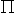rules which are available in the system. These tell you the sort of a dependent product type depending on the sorts of its domain and range types.

# Axes:

 The axes provide a coordinate system spatially locating the systems according which instances of therules are available in each system.
The (*,*) rule, which asserts that the types are closed under the (dependent) function space constructor, is common to all eight systems, and so might be thought of as labelling the origin.

Three other pairs appear, each of which corresponds to an axis of the coordinate system.

Face Without Axis Face With
"Standard" Models (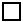,*) Types dependent on Kinds 2
(,) Kinds Dependent on Kinds w(*,) Kinds Dependent on Types P

# Faces:

(,*)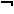(*,)(,) (*,) (,)(,*)

# Vertices:

 (,*) (,) (*,*)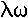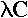(,*) (,) (*,*) (*,)
 (,*) (*,*)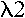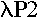(,*) (*,*) (*,)
 (,) (*,*)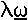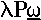(,) (*,*) (*,)
 (*,*)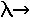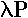(*,*) (*,)

#The Simply Typed Lambda Calculus

CoordinatesPw2
(*,*)
This is said by Barendregt to correspond to Church's simply typed lambda calculus, but that system does not admit dependent types. It is also sometimes alleged to be "hol", possibly meaning by that the logical system supported by the Cambridge HOL interactive theorem prover. This is even wider off the mark, most importantly because all the systems on the lambda cube can only be construed as logics via the propositions as types paradigm, under which a proof that a term has some type is taken as a proof of a proposition understood to be expressed by the type (in the case of a product type, for example, a proposition quantifying over the domain of the product and asserting over that domain the proposition corresponding to the type of the codomain). However, the logic commonly called HOL is a polymorphic version (though not the same kind of polymorphism as is found on the lambda-cube) of the classical logic known as Church's Simple Theory of Types, which is obtained from the simply typed lambda calculus by using certain types of terms of the calculus as propositions in a Hilbert-style axiomatic theory. This is logically quite different, and very much stronger than, the system at the origin of the lambda cube.

#Weak Lambda Omega Related to the system POLYREC studied by Renardel de Lafalette .

CoordinatesPw2
(*,*)   (,)

#Polymorphic or Second Order, Typed Lambda Calculus

CoordinatesPw2
(*,*)     (,*)

#The System F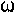CoordinatesPw2
(*,*)   (,) (,*)

#LF Also corresponds to one of the AUTOMATH systems.

CoordinatesPw2
(*,*) (*,)

#Weak Lambda P omega

CoordinatesPw2
(*,*) (*,) (,)

#Lambda P2

CoordinatesPw2
(*,*) (*,)   (,*)

#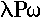The Calculus of Constructions

CoordinatesPw2
(*,*) (*,) (,) (,*)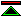©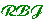created 1995-11-12 modified 2011-05-23